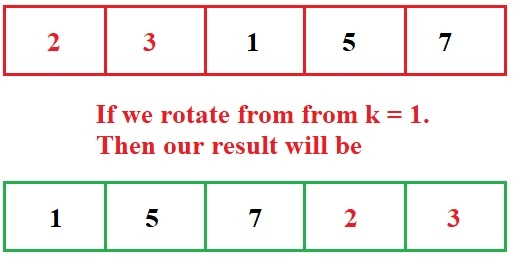# Print left rotation of array in O(n) time and O(1) space in C Program.

We are given an array of some size n and multiple integer values, we need to rotate an array from a given index k.

We want to rotate an array from a index k like −## Examples

Input: arr[] = {1, 2, 3, 4, 5}
K1 = 1
K2 = 3
K3 = 6
Output:
2 3 4 5 1
4 5 1 2 3
2 3 4 5 1

## Algorithm

START
Step 1 -> Declare function void leftRotate(int arr[], int n, int k)
Declare int cal = k% n
Loop For int i=0 and i<n and i++
Print arr[(cal+i)%n]
End
Step 2 -> In main()
Declare array a[]={ 1,2,3,4}
Declare int size=sizeof(a)/sizeof(a)
Declare int k=1
Call leftRotate(a, size, k)
Set k=2
Call leftRotate(a, size, k)
Set k=3
leftRotate(a, size, k)
STOP

## Example

#include <bits/stdc++.h>
using namespace std;
void leftRotate(int arr[], int n, int k){
int cal = k % n;
for (int i = 0; i < n; i++)
cout << (arr[(cal + i) % n]) << " ";
cout << "";
}
int main(){
int a[] = { 1,2,3,4};
int size = sizeof(a) / sizeof(a);
int k = 1;
leftRotate(a, size, k);
k = 2;
leftRotate(a, size, k);
k = 3;
leftRotate(a, size, k);
return 0;
}

## Output

if we run above program then it will generate following output

2 3 4 1
3 4 1 2
4 1 2 3